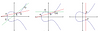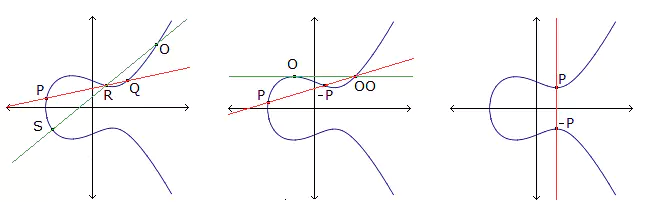# Multiple coverings of the farthest-off points with small density from projective geometry

### Journal article

D. Bartoli, A. Davydov, M. Giulietti, S. Marcugini, F. Pambianco

Semantic Scholar DBLP DOI
Cite

### Cite

APA
Bartoli, D., Davydov, A., Giulietti, M., Marcugini, S., & Pambianco, F. (2015). Multiple coverings of the farthest-off points with small density from projective geometry. Adv. Math. Commun.

Chicago/Turabian
Bartoli, D., A. Davydov, M. Giulietti, S. Marcugini, and F. Pambianco. “Multiple Coverings of the Farthest-off Points with Small Density from Projective Geometry.” Adv. Math. Commun. (2015).

MLA
Bartoli, D., et al. “Multiple Coverings of the Farthest-off Points with Small Density from Projective Geometry.” Adv. Math. Commun., 2015.

## Abstract

In this paper we deal with the special class of covering codes consisting of multiple coverings of the farthest-off points (MCF). In order to measure the quality of an MCF code, we use a natural extension of the notion of density for ordinary covering codes, that is the $\mu$-density for MCF codes; a generalization of the length function for linear covering codes is also introduced. Our main results consist in a number of upper bounds on such a length function, obtained through explicit constructions, especially for the case of covering radius $R=2$. A key tool is the possibility of computing the $\mu$-length function in terms of Projective Geometry over finite fields. In fact, linear $(R,\mu )$-MCF codes with parameters $[n,n-r,d]_{q}R$ have a geometrical counterpart consisting of special subsets of $n$ points in the projective space $PG(n-r-1,q)$. We introduce such objects under the name of $(\rho,\mu)$-saturating sets and we provide a number of example and existence results. Finally, Almost Perfect MCF (APMCF) codes, that is codes for which each word at distance $R$ from the code belongs to {exactly} $\mu$ spheres centered in codewords, are considered and their connections with uniformly packed codes, two-weight codes, and subgroups of Singer groups are pointed out.

Share